# 6 Soliton characteristics

Having explored the existence domains of electron-acoustic solitons, subject to the constraints of the plasma model, we now turn to consider aspects of the soliton characteristics. We have numerically solved Eq. (32) for different representative parameter values, in order to investigate their effects on the soliton characteristics. We point out that, varying different parameters, we have found only negative potential solitons, regardless of the value of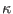considered. This is not altogether unexpected, as it has been found in a number of examples that, in contrast to the Cairns model, , the kappa distribution does not lead to reverse polarity acoustic solitons. [20,47]

Figure 4 shows the variation of the Sagdeev pseudopotential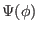with the normalized potential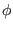, along with the associated pulse solutions (soliton profiles), for different values of the temperature ratio,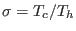(keeping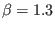,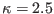and Mach number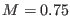, all fixed). The Sagdeev potential well becomes deeper and wider as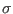is increased. We thus find associated increases in the soliton amplitude and in profile steepness (see Fig. 4b). Thermal effects therefore are seen to amplify significantly the electric potential disturbance at fixed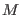.

Figure 5a shows the Sagdeev pseudopotentialfor different values of. The electrostatic pulse (soliton) solution depicted in Fig. 5b is obtained via numerical integration. The pulse amplitude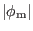increases for lower, implying an amplification of the electric potential disturbance as one departs from the Maxwellian. Once the electric potential has been obtained numerically, the cool-electron fluid density (Fig. 5c) and velocity disturbances (Fig. 5d) are determined algebraically. Both these disturbances are positive in this case, and again, for lowervalues, the profiles reflecting the compression and the increase in velocity are steeper but narrower.

We recall that as various parameter values are varied, the true acoustic speed in the plasma configuration,, also varies. As solitons are inherently super-acoustic, it is clear that the effect of a changing true acoustic speed could mask other dependences. Hence it is also desirable to explore soliton characteristics as a function of the propagation speed, measured relative to the true acoustic speed,. This ratio,, thus represents the “true" Mach number. It has been shown that for any plasma made up of barotropic fluids, arbitrary amplitude solitons satisfy, [48,49,50] from which it follows that, whereis the soliton amplitude. Thus one expects that the soliton amplitude is an increasing function of. This is true for both KdV solitons (small amplitudes, propagating near the sound speed) - “taller is faster”- and, in principle, also for fully nonlinear (Sagdeev) pulses (where the soliton characteristics can only be found numerically. [47,51]) In Fig. 6, we have plotted the soliton amplitudeas a function of the ratio, for a range of values of the parameter. Clearly, the amplitude increases linearly withfor all values of. The two plots for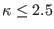both cover the full range up to. However, although we deduce from earlier figures thatincreases with, we see that the endpoints of the plots foroccur at decreasing values of, and indeed decreasing maximum amplitudes. That occurs as, for the chosen values of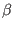and,exceeds unity for, and we have truncated the curves at the point where, to remain within the range defined by the plasma model.

The effect of the hot-to-cool electron density ratio,on the soliton characteristics is shown in Fig. 7. We see that the soliton excitations are amplified and profiles steepened (the Sagdeev potential well becomes wider and deeper), as the density of the hot (nonthermal) electrons is increased (i.e., for higher), viz., keeping,andfixed. Furthermore, an increase in the number density of the hot electrons also leads to an increase in the perturbation of both density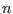, and velocity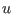of the cool electrons (figure omitted).

Ashkbiz Danehkar
2018-03-28